## Sunday, 13 January 2013

### CBSE Class 9 - Science - CH 12 Sound (MCQs)

SOUND
MCQs

Q1: Which of the following waves do not require any kind of medium to propagate?

(a) Sound
(b) Earthquake waves
(c) Light waves
(d) Water surface waves

Q2: Waves produced on the surface of water are:

(a) Longitudinal only
(b) Transverse waves only
(c) Electromagnetic Waves
(d) Both Longitudinal and Transversal

Q3: Propagation of wave transfers:

(a) energy only
(b) matter only
(c) both energy and matter
(d) none of these

Q4: In longitudinal waves, the particles vibrate in a ___________ direction of propagation

(a) parallel
(b) perpendicular
(c) curved
(d) both parallel and perpendicular

Q5: A medium transmits a sound wave by virtue of its?

(a) elasticity
(b) inertia
(c) density
(d) both inertia and elasticity

Q6: The number of oscillations completed in one second is called _________.

(a) time period
(b) velocity
(c) frequency
(d) wavelength

Q7: What type of sound waves can propagate in solids ?

(a) Longitudinal only
(b) Transverse only
(c) Either longitudinal or transverse
(d) Non mechanical waves only

Q8:The time taken to complete one oscillation is called _________.

(a) time period
(b) velocity
(c) frequency
(d) wavelength

Q9: The period of a vibrating body of frequency 100Hz is

(a) 100 second
(b) 10 second
(c) 0.1 second
(d) 0.01 second

Q10: The SI unit of wavelength is

(a) metre
(b) s-1
(c) metre/second
(d) Hertz

Q11: The quantity 1/λ is known as

(a) wave velocity
(b) frequency
(c) wavelength
(d) wave number

Q12: Sound wave of which of the following frequency is an ultrasonics sound?

(a) 30 Hz
(b) 300 Hz
(c) 3000 Hz
(d) 30,000 Hz

Q13: In which of the following, speed of the sound is maximum:

(a) air
(b) water
(c) steel
(d) kerosene

Q14: When echo is heard ?

(a) If the time interval between original sound and reflected sound is more than 1/10s.
(b) If the time interval between original sound and reflected sound is less 1/10s.
(c) If the tme interval between original sound and reflected sound is less than 1/120s.
(d) If the time interval between original sound and reflected sound is 1/120s

Sound Quiz also available on eduvictors.com
Worksheet on Sound

1: (c) Light waves
2: (b) Transverse waves only
3: (a) energy only
4: (a) parallel
5: (c) density
6: (c) frequency
7: (c) Either longitudinal or transverse
8: (a) time period
9: (d) 0.01 second
10: (a) metre
11: (d) wave number
12: (d) 30000 Hz
13: (c) steel
14: (a) If the time interval between original sound and reflected sound is more than 1/10s.1.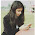Really good thanks :D

1.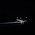You r dumbess

2.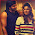useful....helped me n xam :) !i

3.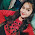more mcq publish

4.Thanks for publishing this mcq please publish more mcq

5.6.Very useful for competative exams.....

7.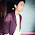Very important question

8.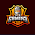Very useful it's can make easy to competition

9.Help me in xam I’m veri pourrr